Calorimetry (2018 NHT) 1) The energy content of foods can be determined using a bomb calorimeter similar to the one shown in the diagram below.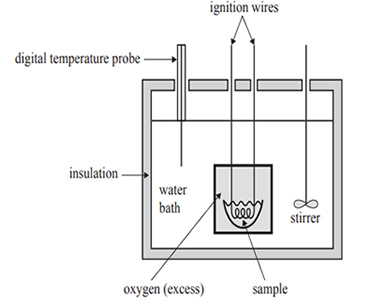The calibration factor for the bomb calorimeter is initially determined by burning a known amount of naphthalene, C10H8. The combustion reaction for C10H8 is shown below.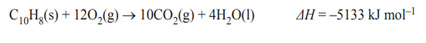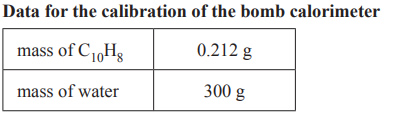The graph produced by the digital temperature probe in the bomb calorimeter is shown below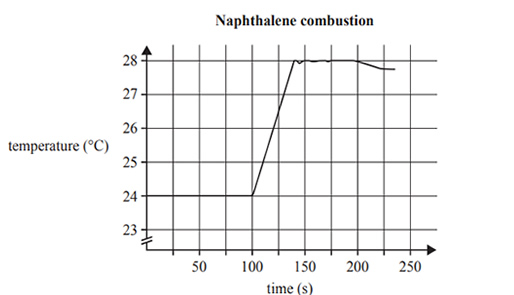a) Use the data in the graph above right, to calculate the calibration factor for the bomb calorimeter. b) Comment on the reliability of the data collected using this bomb calorimeter. Solution3) 2.70 g of a cereal is burnt in a different bomb calorimeter. Below is an extract from the nutrition label on the cereal package.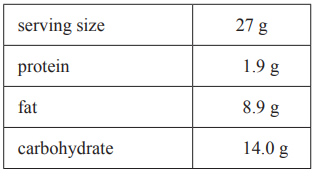i. Calculate the total amount of energy released from the complete combustion of the 2.70 g sample of cereal. ii. The bomb calorimeter used has a calibration factor of 3.3 kJ °C–1. Calculate the maximum expected temperature change in this bomb calorimeter. Solution